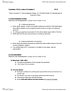# CHEM 1127Q Lecture Notes - Lecture 6: Royal Aircraft Factory F.E.2, Iron(Iii) Oxide, Ammonium Sulfate

60 views3 pageskhangpham8412 and 36530 others unlocked44
Verified Note
44 documents

## Document Summary

Lecture 6 - chapter 3: composition of substances and solutions. Moles are # of molecules in a substance. Great for lab, not great for calculations. Moles and # of atoms are great for relating products to reactants. Mass = molar mass (g/mole) x n (# of moles) Molar masses - na = 22. 99 g/mole, cl= 35. 25 g/mole. Mm= ( 22. 99 g/mole * 1 mol) + ( 35. 25 g/mole * 1 mol) Molar masses - h = 1. 008 g/mole, o = 15. 99 g/mole, s = 32. 065. Mm= ( 1. 008 g/mole * 2 mol) + ( 15. 99 g/mole * 4 mol) + ( 32. 065. How to find moles from molecular formula g/mole * 1 mol) g/mole. C = 12. 011 g/mole, h = 1. 008 g/mole, o=15. 99 g/mole. = 1(14. 01 g/mole) +4(1. 008 g/mole) = 18. 042 g/mol. 2- = 1(32. 06 g/mol) +4(16. 00 g/mole) = 96. 06 g/mole. Total mass = mass cation + mass anion.